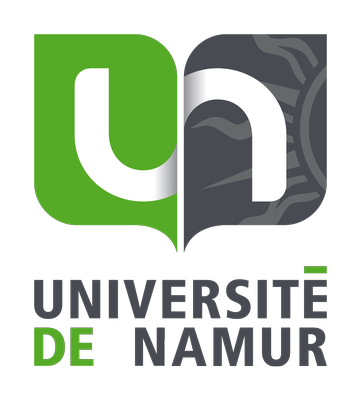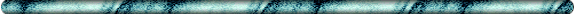## Alexandre Mayer Sélection de publications représentatives1. A. Mayer and J.-P. Vigneron, Transfer matrices combined with Green's functions for the multiple-scattering simulation of electronic projection imaging, Physical Review B 60, 2875 (1999)
2. This paper describes the methodology used for the simulation of the Fresnel projection microscope. The transfer-matrix formalism is used to compute the electronic scattering between the metal supporting the field-emission tip and the anode supporting the sample. The wave function is then propagated, within the Green's-functions formalism, from this anode to a distant screen. This full-order technique is applied to the simulated observation of a carbon fiber containing heterogeneities.

3. A. Mayer and J.-P. Vigneron, Accuracy-control techniques applied to stable transfer-matrix computations, Physical Review E 59, 4659 (1999)
4. This paper describes a key aspect of the transfer-matrix technique: the control of stability. The numerical instabilities are controlled by dividing the cathode-anode distance into adjacent layers and applying the layer addition algorithm. The paper enables one to predict the number of subdivisions to consider as well as the relative error on the final result. It also gives the tools required for an effective calculation of the relative error on the transfer-matrix computation.

5. A. Mayer and J.-P. Vigneron, Non-square transfer-matrix technique applied to the simulation of electronic diffraction by a three-dimensional circular aperture, Physical Review E 61, 5953 (2000)
6. This paper describes an extension of the transfer-matrix formalism to consider non-square matrices. These non-square matrices appear each time the number of basis states used for the representation of the wave function in the region of incidence is different from that used in the region of transmission. The reason for this difference is usually the constant potential energy in these two regions being at different levels or the representation of the wave function in the intermediate region (which contains the diffusers) requiring more components.

7. A. Mayer and J.-P. Vigneron, Group theory used to improve the efficiency of transfer-matrix computations, Physical Review E 60, 7533 (1999)
8. This is a technical paper enabling a drastic reduction of the computational requirements of a transfer-matrix calculation if symmetries are present (in the case of a Cn symmetry axis, the storage space requirements are divided by n3 and the computation time by n2). When the potential energy is real-valued, the basis states appear in pairs of conjugated sets and the transfer matrices associated with one set can be derived immediately from the transfer matrices associated with the other, thus reducing the total computation time by an additional factor of 2.

9. A. Mayer and J.-P. Vigneron, Inverse electronic scattering by Green's functions and singular values decomposition, Physical Review B 62, 16023 (2000)
10. This paper describes a full-order reconstruction scheme, which is suited to the analysis of Fresnel diffraction patterns obtained by low-energy projection microscopy. The method relies on the Green's-functions formalism and singular values decomposition techniques. It enables an iterative reconstruction of the wave function in the sample and on the observation screen and provides in a final step the potential energy in the sample. The technique is applied to the reconstruction of a flat sample and is proved stable against random noise.

11. A. Mayer Band structure and transport properties of carbon nanotubes using a local pseudopotential and a transfer-matrix technique, Carbon 42, 2057 (2004)
12. This paper describes a local pseudopotential that is representative of sp2 carbon atoms in graphene and graphite. It is used for band-structure and transport calculations in carbon nanotubes by using the transfer-matrix methodology.

13. A. Mayer Formulation in terms of normalized propagators of a charge-dipole model enabling the calculation of the polarization properties of carbon nanotubes and fullerenes, Physical Review B 75, 045407 (2007)
14. This paper presents a model for the calculation of molecular polarizabilities in which each atom of the structure considered is represented by a net electric charge and by a dipole. The consideration of electric charges improves the modeling of structures in which electrons are free to move from one atomic site to another. This model is essentially an extension of previous models, which account for dipoles only. Its formulation in terms of normalized propagators improves the stability of the algorithm.

15. A. Mayer, M.S. Chung, B.L. Weiss, N.M. Miskovsky and P.H. Cutler Three-dimensional analysis of the rectifying properties of geometrically asymmetric metal-vacuum-metal junctions treated as an oscillating barrier, Physical Review B 78, 205404 (2008)
16. This paper presents a transfer-matrix methodology for the simulation of electronic scattering in metal-vacuum-metal junctions that are subject to an oscillating bias. The formalism accounts for three-dimensional aspects of the potential energy in the junction as well as for the time-dependence of the problem explicitly. The paper demonstrates that the rectification of optical frequencies can be achieved by using geometrically asymmetric metal-vacuum-metal junctions.

17. A. Mayer, M.S. Chung, P.B. Lerner, B.L. Weiss, N.M. Miskovsky and P.H. Cutler Classical and quantum responsivities of geometrically asymmetric metal-vacuum-metal junctions used for the rectification of infrared and optical radiations, Journal of Vacuum Science & Technology B 29, 041802 (2011)
18. This paper determines the classical responsivity of geometrically asymmetric metal-vacuum-metal junctions. The results are obtained by a classical analysis of static I-V data (quasi-static approximation valid in the infrared). The results are then compared with those provided by a full quantum-mechanical treatment in which the time-dependence of the problem is considered explicitly. Tbis work paves the way to the development of ultra-fast diodes for the harvesting of radiations with frequencies between 1 and 1000 THz.

19. A. Mayer, A. Bay, L. Gaouyat, D. Nicolay, T. Carletti and O. Deparis Genetic algorithms used for the optimization of light-emitting diodes and solar thermal collectors, Proceedings of SPIE 3987, 918705 (2014)
20. This is a first paper on genetic algorithms. A multi-agent version of the genetic algorithm was developed in order to improve light extraction from a GaN light-emitting diode as well as the efficiency with which flat-plate solar thermal collectors can be used for domestic heating of water. This second application required a multi-objective genetic algorithm since we had to (i) maximize the absorption of solar radiations, and (ii) minimize thermal losses. The genetic algorithm provides in this case a whole list of Pareto-optimal solutions for this problem.